The SIM2D Procedure

Introduction to Spatial Simulation

The purpose of spatial simulation is to produce a set of partial realizations of a spatial random field (SRF)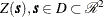in a way that preserves a specified mean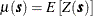and covariance structure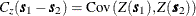. The realizations are partial in the sense that they occur only at a finite set of locations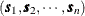. These locations are typically on a regular grid, but they can be arbitrary locations in the plane.

PROC SIM2D produces simulations for continuous processes in two dimensions by using the lower-upper (LU) decomposition method. In these simulations the possible values of the measured quantity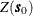at location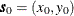can vary continuously over a certain range. An additional assumption, needed for computational purposes, is that the spatial random field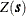is Gaussian. The section Details: SIM2D Procedure provides more information about different types of spatial simulation and associated computational methods.

Spatial simulation is different from spatial prediction, where the emphasis is on predicting a point value at a given grid location. In this sense, spatial prediction is local. In contrast, spatial simulation is global; the emphasis is on the entire realization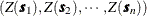.

Given the correct mean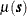and covariance structure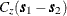, SRF quantities that are difficult or impossible to calculate in a spatial prediction context can easily be approximated by functions of multiple simulations.